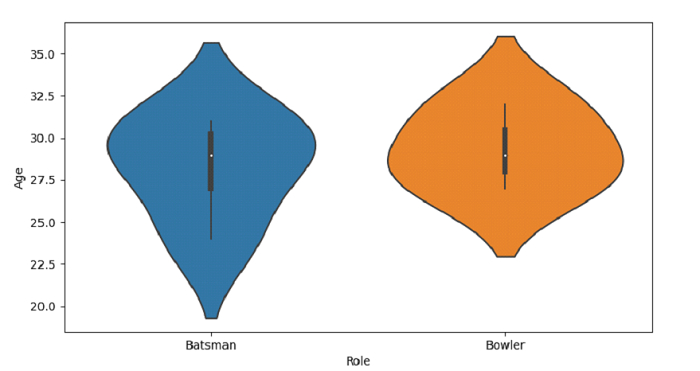# Python Pandas - Draw a violin plot and control order by passing an explicit order with Seaborn

PythonServer Side ProgrammingProgramming

Violin Plot in Seaborn is used to draw a combination of boxplot and kernel density estimate. The seaborn.violinplot() is used for this. Set explicit order using the order parameter of the violinplot().

Let’s say the following is our dataset in the form of a CSV file − Cricketers.csv

At first, import the required libraries −

import seaborn as sb
import pandas as pd
import matplotlib.pyplot as plt

Load data from a CSV file into a Pandas DataFrame −

dataFrame = pd.read_csv("C:\\Users\\amit_\\Desktop\\Cricketers.csv")


Plotting violin plot with Role and Age. Control order by passing an explicit order i.e. ordering on the basis of "Role".

sb.violinplot(x = 'Role', y = "Age", order=["Batsman", "Bowler"], data = dataFrame)

## Example

Following is the code

import seaborn as sb
import pandas as pd
import matplotlib.pyplot as plt

# Load data from a CSV file into a Pandas DataFrame

# plotting violin plot with Role and Age
# Control order by passing an explicit order i.e. ordering on the basis of "Role"
# ordering set using the order parameter
sb.violinplot(x = 'Role', y = "Age", order=["Batsman", "Bowler"], data = dataFrame)

# display
plt.show()

## Output

This will produce the following output −